# 测试开发基础之算法(11):二叉树的三种遍历算法及典型题解

### 1.树和二叉树的核心概念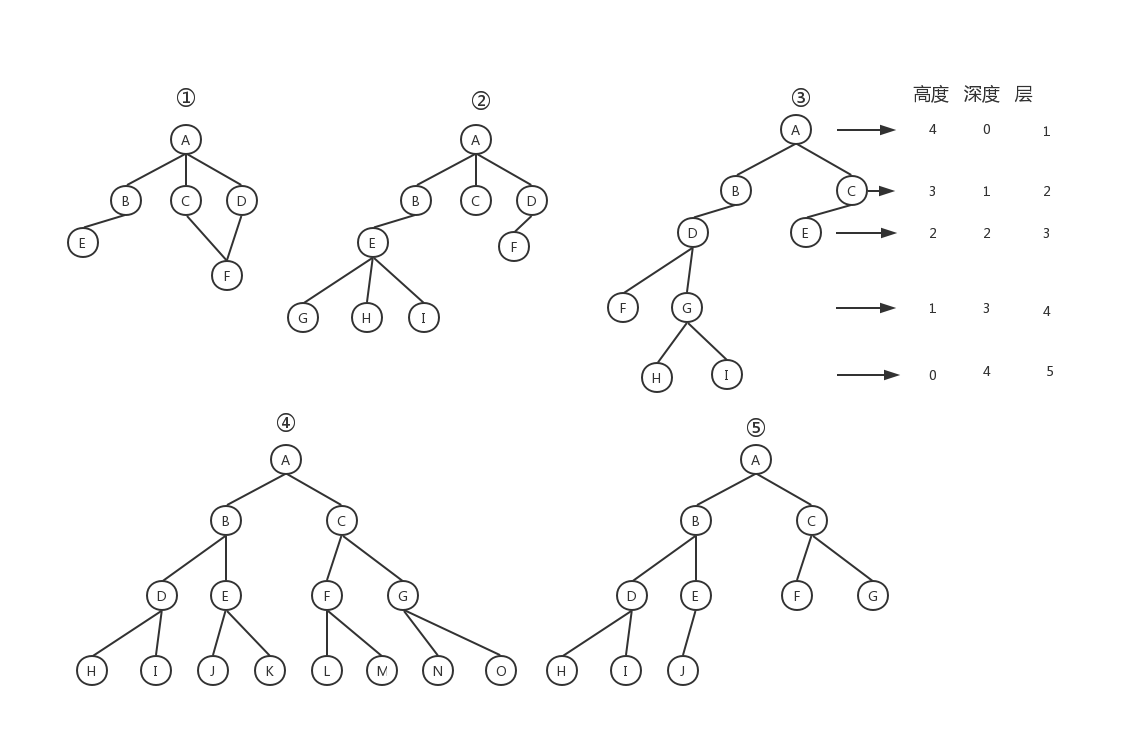“树”还有三个比较相似的概念：高度（Height）、深度（Depth）、层（Level），上面第三幅图展示了三者之间的区别。

### 2.二叉树的存储方式

• 二叉链式存储
二叉链式存储是比较直观、常用和简单的存储法。如下图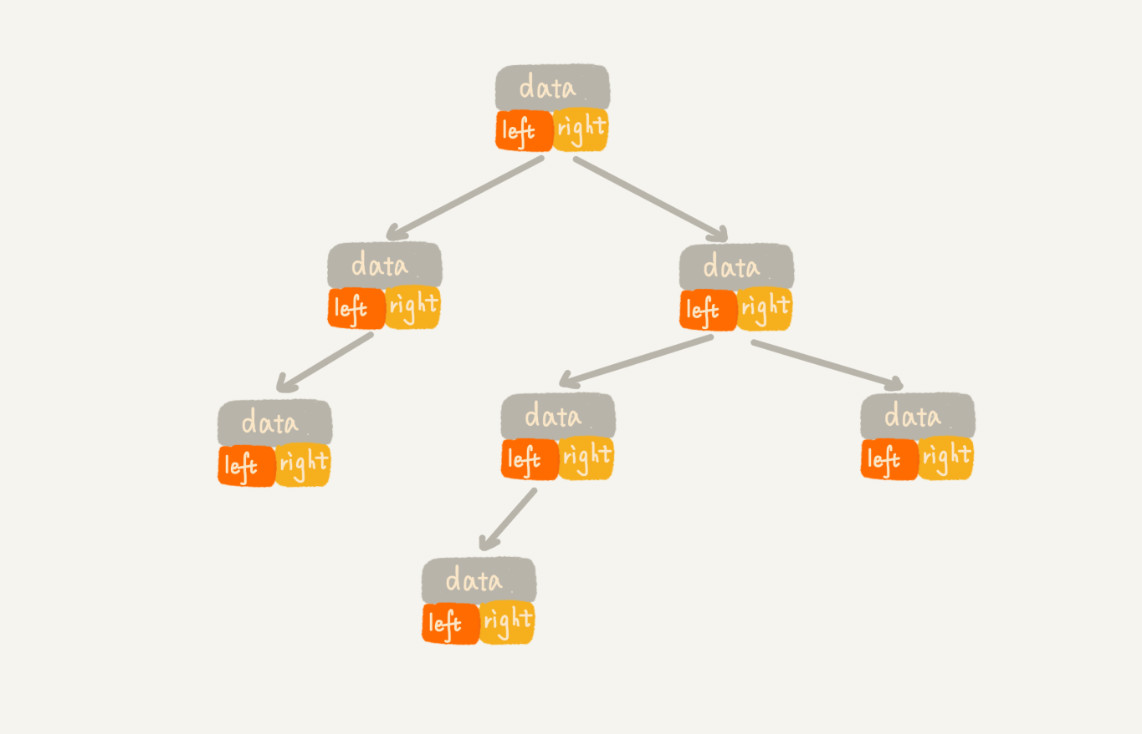树中的每一个字段都有三个字段，分别是数据，左子节点指针和右子节点指针。从根节点开始，使用左右子节点指针，就可以遍历整个树了。

• 数组的顺序存储法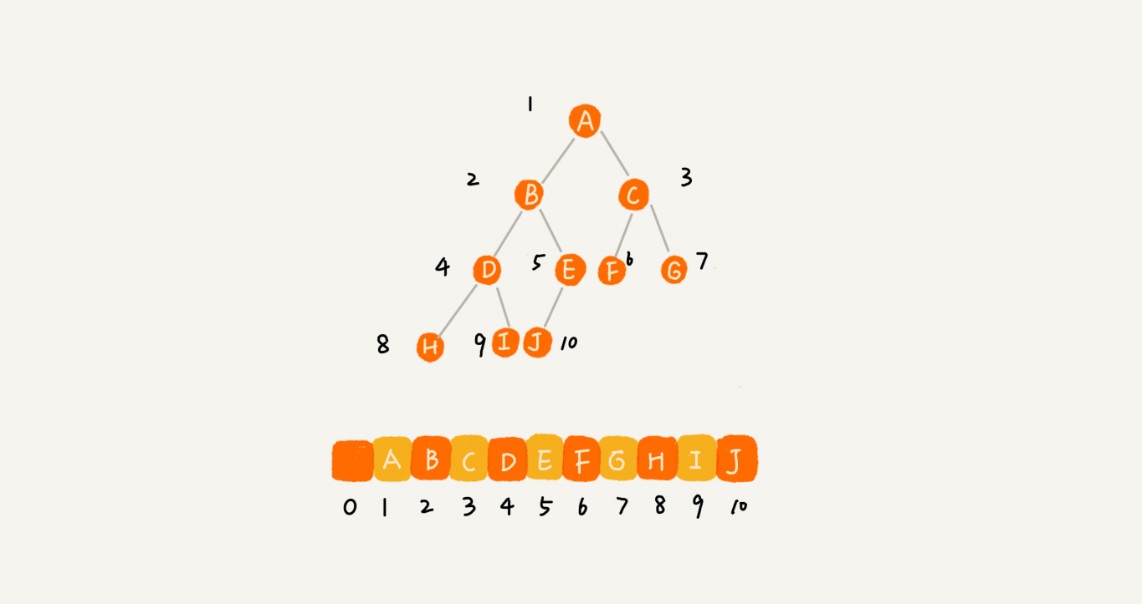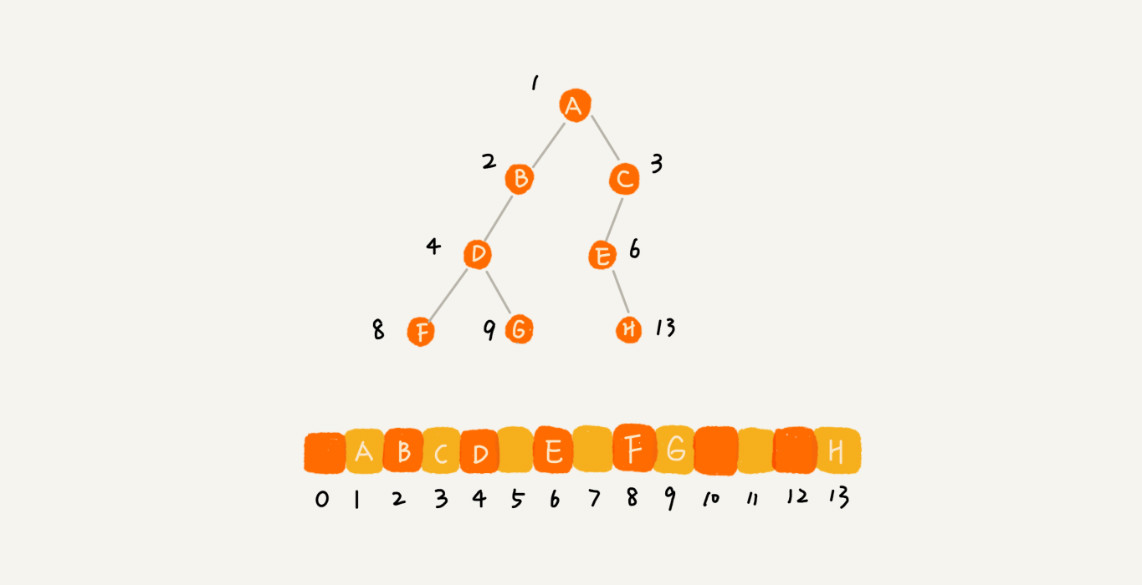### 3.前中后序遍历二叉树

• 前序遍历，指的是先打印当前节点，再打印左子树，最后打印右子树。
• 中序遍历，指的是先打印左子树，再打印当前节点，最后打印右子树。
• 后续遍历，指的是先打印左子树，再打印右子树，最后打印当前节点。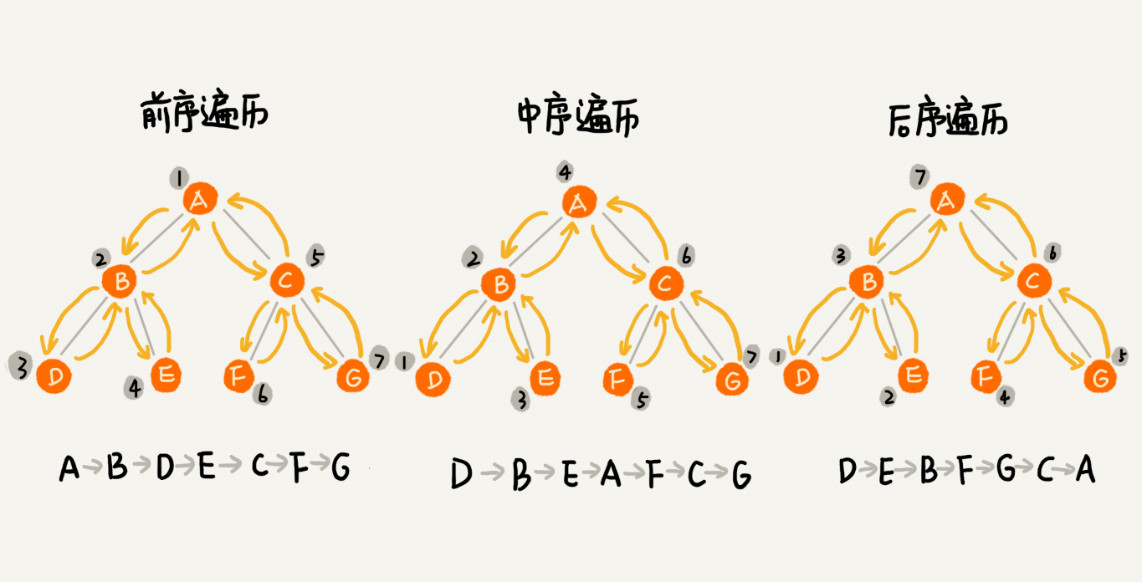preOrder (node) = print node->preOrder(node->left)->preOrder(node->right)

inOrder(node) = inOrder(node->left)->print node->inOrder(node->right)

postOrder(node) = postOrder(node->left)->postOrder(node->right)->print node

from typing import TypeVar, Generic, Optional, Generator

T = TypeVar("T")

class TreeNode(Generic[T]):
def __init__(self, value):
self.value = value
self.left = None
self.right = None

def pre_order(root: Optional[TreeNode[T]]) -> Generator[T, None, None]:
"""
:param root:根节点
:return:生成器
"""
if root:
yield root.value  # 先打印当前节点
yield from pre_order(root.left)  # 再打印左子树
yield from pre_order(root.right)  # 再打印右子树

def in_order(root: Optional[TreeNode[T]]) -> Generator[T, None, None]:
if root:
yield from in_order(root.left)
yield root.value
yield from in_order(root.right)

def post_order(root: Optional[TreeNode[T]]) -> Generator[T, None, None]:
if root:
yield from post_order(root.left)
yield from post_order(root.right)
yield root.value

if __name__ == '__main__':
b_tree = TreeNode("root_node")

second_layer_left = TreeNode("second_layer_left")
second_layer_right = TreeNode("second_layer_right")

first = TreeNode("first")
second = TreeNode("second")
third = TreeNode("third")
fourth = TreeNode("fourth")
fifth = TreeNode("fifth")
sixth = TreeNode("sixth")

# 拼接成树
b_tree.left = second_layer_left
b_tree.right = second_layer_right

second_layer_left.left = first
second_layer_left.right = second

second_layer_right.left = third
second_layer_right.right = fourth

first.left = fifth
second.left = sixth

# 前序遍历
print(list(pre_order(b_tree)))
# 中序遍历
print(list(in_order(b_tree)))
# 后续遍历
print(list(post_order(b_tree)))


### 4.按层遍历二叉树

    3
/ \
9  20
/  \
15   7


[
,
[9,20],
[15,7]
]


def layer_order(root: Optional[TreeNode[T]]) -> list:
layers = []  # 输出结果列表

def layer_order_dfs(node, layer):
if not node:  # 递归终止条件
return layers

# 处理当前层
if len(layers) <= layer:  # 当前节点所在层大于等于输出列表长度时，给当前节点所在层新创建一个列表
layers.append([])
layers[layer].append(node.value)  # 当前节点加入当前层的列表中

# 递归处理下一层
layer_order_dfs(node.left, layer + 1)
layer_order_dfs(node.right, layer + 1)

layer_order_dfs(root, 0)  # 从第1层开始处理
return layers


### 5.练习题：求二叉树最大最小深度

max_depth(node) = max(max_depth(node.left), max_depth(node.right)) + 1

 if node is None:
return 0


def max_depth(node: Optional[TreeNode[T]]) -> int:
if node is None:  # 当输入的节点为空节点时，我们无需继续计算其子树的深度，直接返回0，结束递归函数
return 0
if node.right is None:  # 当前节点不为空，但是右子树为空，直接返回左子树的最大深度
return max_depth(node.left) + 1
if node.left is None:  # 当前结点不为空，右子树不为空，但是左子树为空，直接返回右子树的最大深度
return max_depth(node.right) + 1
# 当前节点不为空，左右子节点也不为空，返回左右子树最大深度
return max(max_depth(node.left), max_depth(node.right)) + 1


def min_depth(node: Optional[TreeNode[T]]) -> int:
if node is None:
return 0
if node.left is None:
return min_depth(node.right) + 1
if node.right is None:
return min_depth(node.left) + 1
return min(min_depth(node.left), min_depth(node.right)) + 105-255万+
12-0442
09-271万+
10-193125
08-2925万+
03-071071
08-203147
11-101万+
11-10531
01-172万+
10-111万+
08-27205
12-15402
03-201万+
07-134544
05-234205
07-041303
02-2118万+
©️2020 CSDN 皮肤主题: 编程工作室 设计师:CSDN官方博客